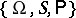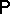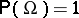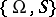# Probability distribution

(diff) ← Older revision | Latest revision (diff) | Newer revision → (diff)

One of the basic concepts in probability theory and mathematical statistics. In the modern approach, a suitable probability spaceis taken as a model of a stochastic phenomenon being considered. Hereis a sample space,is a-algebra of subsets ofspecified in some way andis a measure onsuch that(a probability measure).

Any such measure onis called a probability distribution (see ). But this definition, basic in the axiomatics introduced by A.N. Kolmogorov in 1933, proved to be too general in the course of the further development of the theory and was replaced by more restrictive ones in order to exclude some "pathological" cases. An example was the requirement that the measurebe "perfect" (see ). Probability distributions in function spaces are usually required to satisfy some regularity property, usually formulated as separability but also admitting a characterization in different terms (see Separable process and also ).

Many of the probability distributions that appear in the specific problems in probability theory and mathematical statistics have been known for a long time and are connected with the basic probability schemes . They are described either by probabilities of discrete values (see Discrete distribution) or by probability densities (see Continuous distribution). There are also tables compiled in certain cases where they are necessary .

Among the basic probability distributions, some are connected with sequences of independent trials (see Binomial distribution; Geometric distribution; Multinomial distribution) and others with the limit laws corresponding to such a probability scheme when the number of trials increases indefinitely (see Normal distribution; Poisson distribution; Arcsine distribution). But these limit distributions may also appear directly in exact form, as in the theory of stochastic processes (see Wiener process; Poisson process), or as solutions of certain equations arising in so-called characterization theorems (see also Normal distribution; Exponential distribution). A uniform distribution, usually considered as a mathematical way of expressing that outcomes of an experiment are equally possible, can also be obtained as a limit distribution (say, by considering sums of large numbers of random variables or some other random variables with sufficiently smooth and "spread out" distributions modulo 1). More probability distributions can be obtained from those mentioned above by means of functional transformations of the corresponding random variables. For example, in mathematical statistics random variables with a normal distribution are used to obtain variables with a "chi-squared" distribution, a non-central "chi-squared" distribution, a Student distribution, a Fisher-distribution, and others.

Important classes of distributions were discovered in connection with asymptotic methods in probability theory and mathematical statistics (see Limit theorems; Stable distribution; Infinitely-divisible distribution; "Omega-squared" distribution).

It is important, both for the theory and in applications, to be able to define a concept of proximity of distributions. The collection of all probability distributions oncan in different ways be turned into a topological space. Weak convergence of probability distributions plays a basic role here (see Distributions, convergence of). In the one-dimensional and finite-dimensional cases the apparatus of characteristic functions (cf. Characteristic function) is a principal instrument for studying convergence of probability distributions.

A complete description of a probability distribution (say, by means of the density of a probability distribution or a distribution function) is often replaced by a limited collection of characteristics. The most widely used of these in the one-dimensional case are the mathematical expectation (the average value), the dispersion (or variance), the median (in statistics), and the moments (cf. Moment). For numerical characteristics of multivariate probability distributions see Correlation (in statistics); Regression.

The statistical analogue of a probability distribution is an empirical distribution. An empirical distribution and its characteristics can be used for the approximate representation of a theoretical distribution and its characteristics (see Statistical estimator). For ways to measure how well an empirical distribution fits a hypothetical one see Statistical hypotheses, verification of; Non-parametric methods in statistics.

How to Cite This Entry:
Probability distribution. Encyclopedia of Mathematics. URL: http://encyclopediaofmath.org/index.php?title=Probability_distribution&oldid=13959
This article was adapted from an original article by Yu.V. Prokhorov (originator), which appeared in Encyclopedia of Mathematics - ISBN 1402006098. See original article